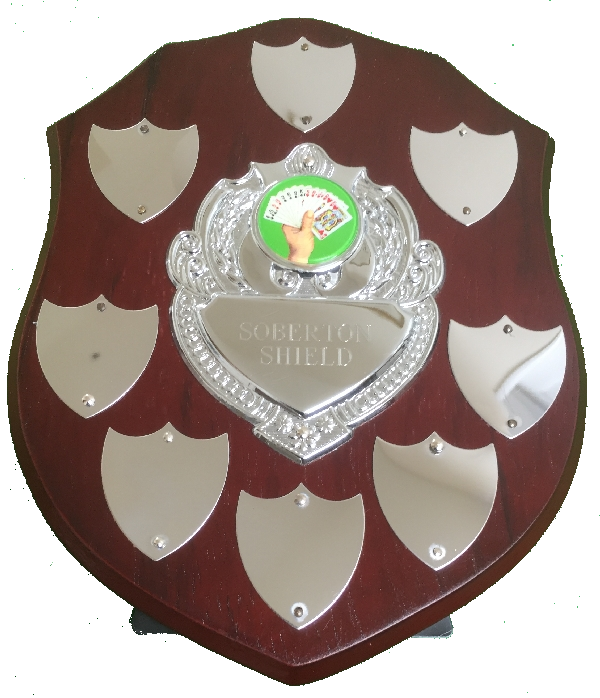# Soberton Shield Results - 2018

Team Jan 5 Feb 9 Apr 13 May 11 Oct 5 Nov 2 Total
Lord, Ladies And Gentlemen (1) 25 +12 = 37 5 -4 = 1 5 +24 = 29 -6 +4 = -2 -21 +4 = -17 7 +4 = 11 59
Five Queens And A Knave (2) -5 +32 = 27 -78 +12 = -66 -43 +20 = -23 14 +16 = 30 33 +32 = 65 -47 +16 = -31 2
Six Hearts (3) 18 -8 = 10 29 -12 = 17 16 +8 = 24 -9 -8 = -17 19 -12 = 7 42 +4 = 46 87
Overcallers (4) -47 +32 = -15 11 +20 = 31 -16 +24 = 8 -14 -12 = -26 -36 +20 = -16 65 -20 = 45 27
Acolytes (5) 43 -12 = 31 56 +0 = 56 4 -4 = 0 14 +0 = 14 11 -8 = 3 9 -8 = 1 105
Ruffs (6) -14 +20 = 6 8 +16 = 24 36 +0 = 36 -4 +28 = 24 8 +20 = 28 -6 +20 = 14 132
Waltham Wanderers (7) -21 +8 = -13 -59 +4 = -55 -16 +16 = 0 15 -8 = 7 -37 -4 = -41 2 +12 = 14 -88
Sparkling Diamonds (8) -49 +24 = -25 -36 +20 = -16 6 +32 = 38 -47 +20 = -27 -16 +12 = -4 -69 +20 = -49 -83
Mismatched (9) 54 -12 = 42 64 -28 = 36 8 +4 = 12 37 -20 = 17 2 +8 = 10 -3 +4 = 1 118
Total IMPs 140 - 136 = 4 173 - 173 = 0 75 - 75 = 0 80 - 80 = 0 73 - 110 = -37 125 - 125 = 0

Under each date for each team is a sum which represents 'IMPs' + 'Handicap' = 'Total'.
The row 'Total IMPs' shows the 'sum of plus scores' - 'the sum of minus scores' = X where X should be zero.

Click on a table heading to sort the column under it.